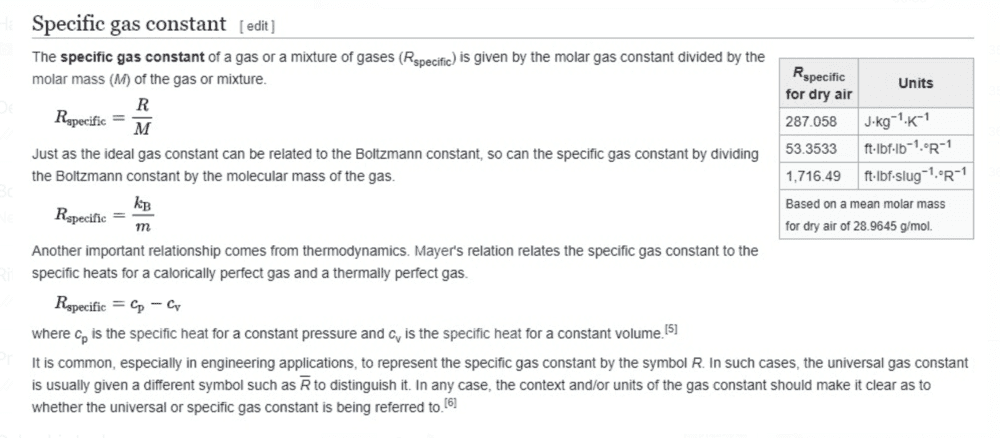# Find the value of Cp using SFEE and Thermodynamic equations

Kajan thana
Homework Statement:
Find the specific heat capacity (Cp) with the given variables:

State:1.... p= 15 bars, Temperature: 600K,
State 2.... p= 1 bar, Temperature : 200, Enthalphy: -2000
This is an isentropic expansion.
Relevant Equations:
Q-W=Cv(t2 -t2)
Cp/Cv= gamma
change in enthalphy = Cp * delta Temperature
I have managed to find the gamma value using the following equation: (T2/T1)= (P2/P1)(gamma-1/gamma. This will give me the answer of 1.29 SF. After this step, I am clueless.

Can someone help me with this question please?
I assume we have to find the enthalpy at state 1 to do that question.

Mentor
This is not the exact statement of the problem. Please provide the exact statement.

•Kajan thana
Kajan thana
This is not the exact statement of the problem. Please provide the exact statement.

Calculate Cp for a perfect gas that undergoes isentropic expansion through a turbine from state 1 (600K, 15bar) to state 2 where the pressure is 1 bar, the temperature is 200K and enthalpy is -2000 kJ/kg (this number is calculated from the previous question) .

Mentor
Calculate Cp for a perfect gas that undergoes isentropic expansion through a turbine from state 1 (600K, 15bar) to state 2 where the pressure is 1 bar, the temperature is 200K and enthalpy is -2000 kJ/kg (this number is calculated from the previous question) .
OK. So the -2000 is irrelevant. What did you get for gamma?

•Kajan thana
Kajan thana
OK. So the -2000 is irrelevant. What did you get for gamma?
gamma= 1.28

Kajan thana
gamma= 1.28
gamma= 1.28
Sorry the gamma is 1.68

Mentor
gamma= 1.28
OK. From that, you should immediately be able to get Cp. How are Cp and Cv related to gamma and how are they related to R?

•Kajan thana
Kajan thana
OK. From that, you should immediately be able to get Cp. How are Cp and Cv related to gamma and how are they related to R?
The relationships are Cp - Cv = R, and Cp / Cv = Gamma. But for this question they haven't mentioned the sort of gas that is used, so we cannot find the gas constant. I'm not sure how to find the Cv or Cp. In order to find the Cv, we need the work done or the change in enthalpy. I'm not sure how to go about doing it. Thanks

Mentor
The relationships are Cp - Cv = R, and Cp / Cv = Gamma. But for this question they haven't mentioned the sort of gas that is used, so we cannot find the gas constant. I'm not sure how to find the Cv or Cp. In order to find the Cv, we need the work done or the change in enthalpy. I'm not sure how to go about doing it. Thanks
You have two equations and 2 unknowns: Cp and Cv. The molar gas constant does not depend on the gas.

Kajan thana
You have two equations and 2 unknowns: Cp and Cv. The molar gas constant does not depend on the gas.
Sorry I am bit confused. I thought that the molar gas constant was not the same as the universal gas constant (8.31). RGAS CONSTANT = R UNIVERSIAL CONSTANT/MOLAR MASS

Mentor
Sorry I am bit confused. I thought that the molar gas constant was not the same as the universal gas constant (8.31). RGAS CONSTANT = R UNIVERSIAL CONSTANT/MOLAR MASS
The molar gas constant is equal to the universal gas constant. The mass gas constant is equal to the universal gas constant divided by the molar mass.

Kajan thana
Sorry I am bit confused. I thought that the molar gas constant was not the same as the universal gas constant (8.31). RGAS CONSTANT = R UNIVERSIAL CONSTANT/MOLAR MASS
THE question doesn't have any information on the gas that is being used like the Molar Mass; apart from the mention of it being a perfect gas.

Mentor
THE question doesn't have any information on the gas that is being used like the Molar Mass; apart from the mention of it being a perfect gas.
Cp they are looking for is the molar value, not the mass value.

Kajan thana
Cp they are looking for is the molar value, not the mass value.
So with the simultaneous equation, I got the answer as 20 kJ/kg/K

Mentor
I get 20.5

•Kajan thana
Kajan thana
I get 20.5
yh I did the calculation wrong
Is there any other way to check the answer?

Kajan thanaKajan thana
I saw this on Wikipedia, where it mentions that Rspecific = Cp -Cv. Is there something that I am misunderstanding?
Thank you.

Mentor
You can express heat capacities per unit mole or per unit mass. The same goes for the gas constant. The gas constant per mole is the universal gas constant.

•Kajan thana
Kajan thana
You can express heat capacities per unit mole or per unit mass. The same goes for the gas constant. The gas constant per mole is the universal gas constant.
Thank you